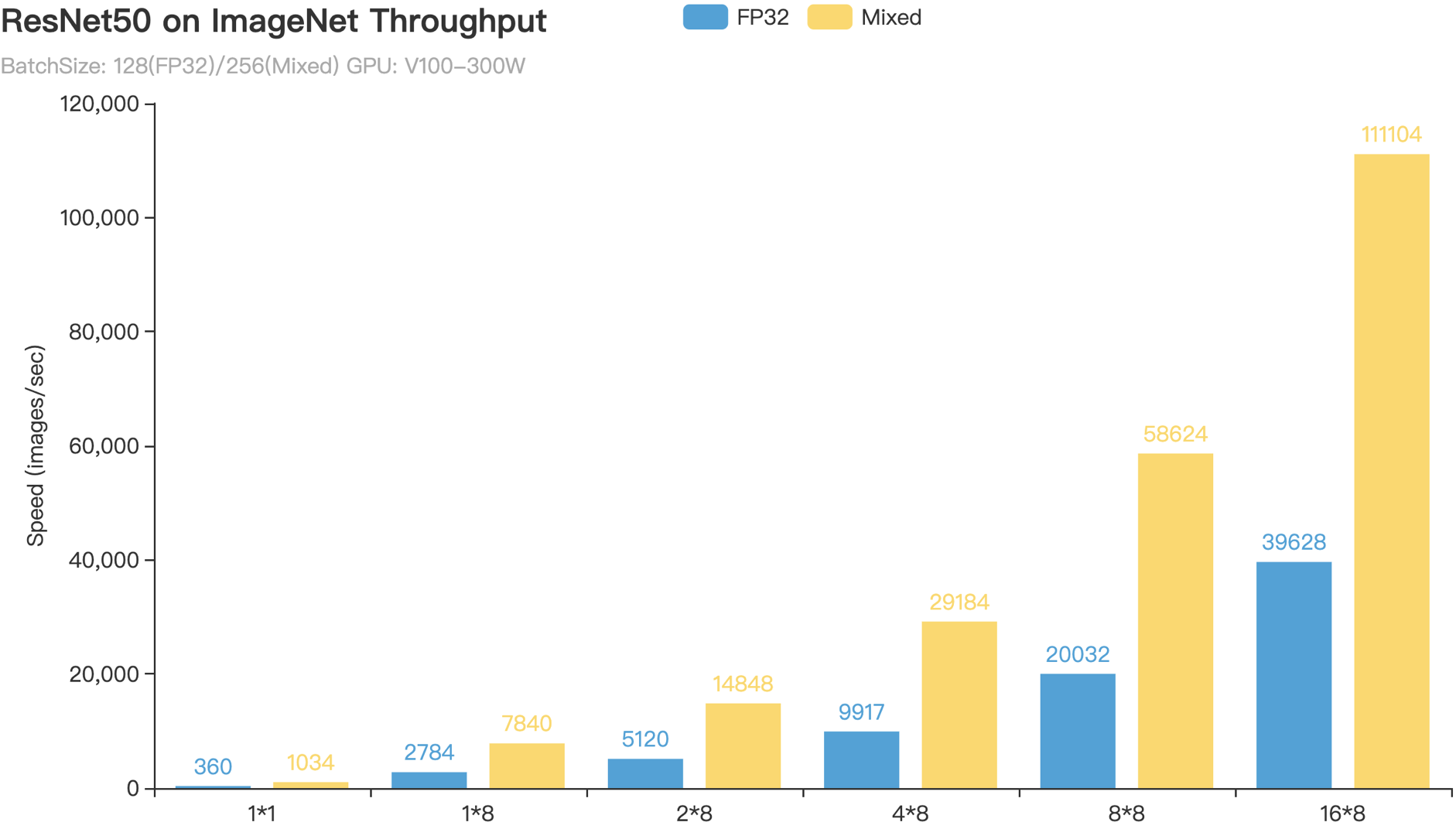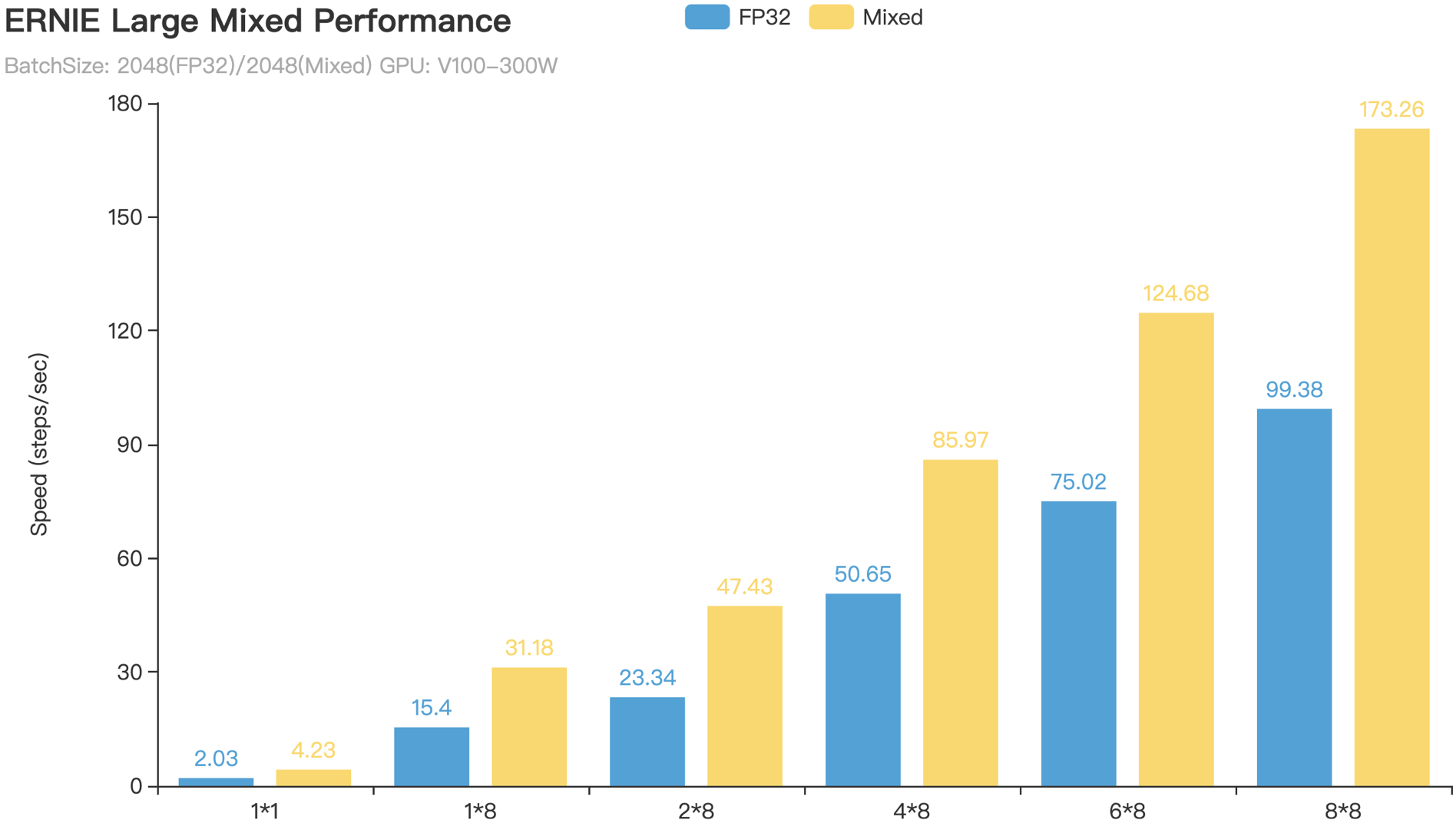# 混合精度训练最佳实践¶

Automatic Mixed Precision (AMP) 是一种自动混合使用半精度（FP16）和单精度（FP32）来加速模型训练的技术。AMP 技术可方便用户快速将使用 FP32 训练的模型修改为使用混合精度训练，并通过黑白名单和动态loss scaling来保证训练时的数值稳定性进而避免梯度 Infinite 或者 NaN(Not a Number)。借力于新一代 NVIDIA GPU 中 Tensor Cores 的计算性能，PaddlePaddle AMP 技术在 ResNet50、Transformer 等模型上训练速度相对于 FP32 训练加速比可达 1.5～2.9。

## 英伟达 GPU 的 FP16 算力¶

• FP16 可降低一半的内存带宽和存储需求，这使得在相同的硬件条件下研究人员可使用更大更复杂的模型以及更大的 batch size 大小。

• FP16 可以充分利用英伟达 Volta 及 Turing 架构 GPU 提供的 Tensor Cores 技术。在相同的 GPU 硬件上，Tensor Cores 的 FP16 计算吞吐量是 FP32 的 8 倍。

MNIST 网络定义

import paddle.fluid as fluid

def MNIST(data, class_dim):
conv1 = fluid.layers.conv2d(data, 16, 5, 1, act=None, data_format='NHWC')
bn1 = fluid.layers.batch_norm(conv1, act='relu', data_layout='NHWC')
pool1 = fluid.layers.pool2d(bn1, 2, 'max', 2, data_format='NHWC')
conv2 = fluid.layers.conv2d(pool1, 64, 5, 1, act=None, data_format='NHWC')
bn2 = fluid.layers.batch_norm(conv2, act='relu', data_layout='NHWC')
pool2 = fluid.layers.pool2d(bn2, 2, 'max', 2, data_format='NHWC')
fc1 = fluid.layers.fc(pool2, size=64, act='relu')
fc2 = fluid.layers.fc(fc1, size=class_dim, act='softmax')
return fc2


• conv2dbatch_norm以及pool2d等需要将数据布局设置为NHWC，这样有助于使用 TensorCore 技术加速计算过程1

• Tensor Cores 要求在使用 FP16 加速卷积运算时 conv2d 的输入/输出通道数为 8 的倍数2，因此设计网络时推荐将 conv2d 层的输入/输出通道数设置为 8 的倍数。

• Tensor Cores 要求在使用 FP16 加速矩阵乘运算时矩阵行数和列数均为 8 的倍数3，因此设计网络时推荐将 fc 层的 size 参数设置为 8 的倍数。

FP32 训练

import paddle
import numpy as np

data = fluid.layers.data(
name='image', shape=[None, 28, 28, 1], dtype='float32')
label = fluid.layers.data(name='label', shape=[None, 1], dtype='int64')

out = MNIST(data, class_dim=10)
loss = fluid.layers.cross_entropy(input=out, label=label)
avg_loss = fluid.layers.mean(loss)

sgd = fluid.optimizer.SGDOptimizer(learning_rate=1e-3)
sgd.minimize(avg_loss)


AMP 训练

sgd = SGDOptimizer(learning_rate=1e-3)
# 此处只需要使用 fluid.contrib.mixed_precision.decorate 将 sgd 封装成 AMP 训练所需的
# 优化器 mp_sgd，并使用 mp_sgd.minimize(avg_loss)代替原来的 sgd.minimize(avg_loss)语句即可。
mp_sgd = fluid.contrib.mixed_precision.decorator.decorate(sgd)
mp_sgd.minimize(avg_loss)


export FLAGS_conv_workspace_size_limit=1024 # MB，根据所使用的 GPU 显存容量及模型特点设置数值，值越大越有可能选择到更快的卷积算法
export FLAGS_cudnn_exhaustive_search=1 # 使用穷举搜索方法来选择快速卷积算法
export FLAGS_cudnn_batchnorm_spatial_persistent=1 # 用于触发 batch_norm 和 relu 的融合


### 自定义黑白名单¶

PaddlePaddle AMP 功能实现中根据 FP16 数据类型计算稳定性和加速效果在框架内部定义了算子（Op）的黑白名单。具体来说，将对 FP16 计算友好且能利用 Tensor Cores 的 Op 归类于白名单，将使用 FP16 计算会导致数值不稳定的 Op 归类于黑名单，将对 FP16 计算没有多少影响的 Op 归类于灰名单。然而，框架开发人员不可能考虑到所有的网络模型情况，尤其是那些特殊场景中使用到的模型。用户可以在使用fluid.contrib.mixed_precision.decorate 函数时通过指定自定义的黑白名单列表来改变默认的 FP16 计算行为。

sgd = SGDOptimizer(learning_rate=1e-3)
# list1 是白名单 op 列表，list2 是黑名单 op 列表，list3 是黑名单 var_name 列表（凡是以这些黑名单 var_name 为输入或输出的 op 均会被视为黑名单 op）
amp_list = AutoMixedPrecisionLists(custom_white_list=list1, custom_black_list=list2, custom_black_varnames=list3)
mp_sgd = fluid.contrib.mixed_precision.decorator.decorate(sgd, amp_list)
mp_sgd.minimize(avg_loss)


### 自动 loss scaling¶

sgd = SGDOptimizer(learning_rate=1e-3)
mp_sgd = fluid.contrib.mixed_precision.decorator.decorate(sgd,
amp_lists=None,
init_loss_scaling=2**8,
incr_every_n_steps=500,
decr_every_n_nan_or_inf=4,
incr_ratio=2.0,
decr_ratio=0.5,
use_dynamic_loss_scaling=True)
mp_sgd.minimize(avg_loss)


init_loss_scaling incr_every_n_steps 以及decr_every_n_nan_or_inf等参数控制着自动 loss scaling 的行为。它们仅当 use_dynamic_loss_scaling设置为 True 时有效。下面详述这些参数的意义：

• init_loss_scaling(float)：初始 loss scaling 值。

• incr_every_n_steps(int)：每经过 incr_every_n_steps 个连续的正常梯度值才会增大 loss scaling 值。

• decr_every_n_nan_or_inf(int)：每经过 decr_every_n_nan_or_inf 个连续的无效梯度值(nan 或者 inf)才会减小 loss scaling 值。

• incr_ratio(float)：每次增大 loss scaling 值的扩增倍数，其为大于 1 的浮点数。

• decr_ratio(float)：每次减小 loss scaling 值的比例系数，其为小于 1 的浮点数。

## 多卡 GPU 训练的优化¶

PaddlePaddle AMP 功能对多卡 GPU 训练进行了深度优化。如图 2 所示，优化之前的参数梯度更新特点：梯度计算时虽然使用的是 FP16 数据类型，但是不同 GPU 卡之间的梯度传输数据类型仍为 FP32。